## Saturday, 9 August 2014

### CHAPTER 4- Worked Out Examples 2

 Example: 4
 (a) What is the greatest coefficient in the expansion of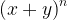${\left( {x + y} \right)^n}$? (b) What is the greatest term in the expression of${\left( {x + y} \right)^n}$?
 Solution: 4-(a)
For this part, we basically need to only determine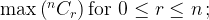$\max \left( {^n{C_r}} \right){\rm{for}}\,\,0 \le r \le n\,;$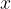$x$ and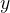$y$ have no role to play in this part.
To find the greatest coefficient, consider the following ratio: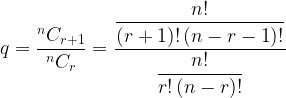$q = \dfrac{{^n{C_{r + 1}}}}{{^n{C_r}}} = \dfrac{{\dfrac{{n!}}{{\left( {r + 1} \right)!\left( {n - r - 1} \right)!}}}}{{\dfrac{{n!}}{{r!\left( {n - r} \right)!}}}}$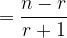$= \dfrac{{n - r}}{{r + 1}}$
Thus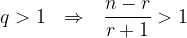$q > 1 \,\,\,\, \Rightarrow \,\,\,\, \dfrac{{n - r}}{{r + 1}} > 1$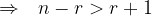$\Rightarrow \,\,\,\, n - r > r + 1$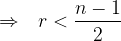$\Rightarrow \,\,\,\, r < \dfrac{{n - 1}}{2}$
Similarly,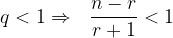$q < 1 \Rightarrow \,\,\,\, \dfrac{{n - r}}{{r + 1}} < 1$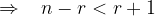$\Rightarrow \,\,\,\,\, n - r < r + 1$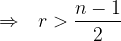$\Rightarrow \,\,\,\, r > \dfrac{{n - 1}}{2}$
Thus,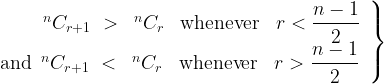$\left. \begin{array}{l} \,\,\,\,\,\,\,\,\,\,\,\,\,{ ^n}{C_{r + 1}} \,\,>\,\, {\,^n}{C_r} \,\,\,\,\, {\rm{whenever}} \,\,\,\,\, r < \dfrac{{n - 1}}{2}\\ {\rm{and}} \,\,\, { ^n}{C_{r + 1}} \,\,< \,\,{\,^n}{C_r}\,\,\,\,\, {\rm{whenever}} \,\,\,\,\,r > \dfrac{{n - 1}}{2}\, \end{array} \right\}$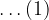$\ldots(1)$
If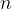$n$ is odd, we have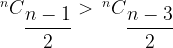$^n{C_{\dfrac{{n - 1}}{2}}} > {\,^n}{C_{\dfrac{{n - 3}}{2}}}$ and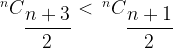$^n{C_{\dfrac{{n + 3}}{2}}} < {\,^n}{C_{\dfrac{{n + 1}}{2}}}$
Also, since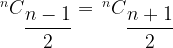$^n{C_{\dfrac{{n - 1}}{2}}} = {\,^n}{C_{\dfrac{{n + 1}}{2}}}$
we see that for odd$n$, the two middle coefficients are the greatest. This can be verified by considering the following expansion:$\begin{array}{l} {\left( {x + y} \right)^5} = {x^5} + 5{x^4}y + \mathop {10}\limits_ \nwarrow {x^3}{y^2} + \mathop {10}\limits_ \nearrow {x^2}{y^3} + 5x{y^4} + {y^5}\\ \,\,\,\,\,\,\,\,\,\,\,\,\,\,\,\,\,\,\,\,\,\,\,\,\,\,\,\,\,\,\,\,\,\, \,\,\,\,\,\,\,{ ^{\scriptstyle\,\,\,\,\,\,\,\,\,\,\,\,{\rm{The}}\,\,{\rm{two}}\,\,{\rm{middle \,coefficients \,are }}\hfill\atop \scriptstyle\,\,\,\,\,\,\,\,\,\,\,\,{\rm{the}}\,\,{\rm{greatest}}\,\,{\rm{for}}\,\,{\rm{odd}}\,\,\,n\hfill}}\,\, \end{array}$
If$n$ is even,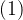$(1)$ gives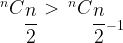$^n{C_{\dfrac{n}{2}}} > {\,^n}{C_{\dfrac{n}{2} - 1}}$ and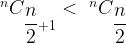$^n{C_{\dfrac{n}{2} + 1}} < \,{\,^n}{C_{\dfrac{n}{2}}}$
In this case therefore, the greatest coefficient is the single middle coefficient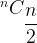$^n{C_{\dfrac{n}{2}}}$. Lets verify this again: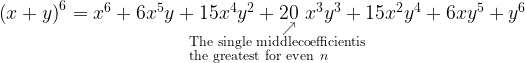$\begin{array}{l} {\left( {x + y} \right)^6} = {x^6} + 6{x^5}y + 15{x^4}{y^2} + \mathop {20}\limits_ \nearrow \,{x^3}{y^3} + 15{x^2}{y^4} + 6x{y^5} + {y^6}\\ \,\,\,\,\,\,\,\,\,\,\,\,\,\,\,\,\,\,\,\,\,\,\,\,\,\,\,\,\,\,\,\,\,\,\,\,\,\,\,\,\,\,\,\,\,\,\,\,\,\,\,\,\,\,{ ^{\scriptstyle\, \,\,\,{\rm{The}}\,\,{\rm{single}}\,\,{\rm{middle coefficient is }}\hfill\atop \scriptstyle\, \,\,\,{\rm{the}}\,\,{\rm{greatest}}\,\,{\rm{for}}\,\,{\rm{even}}\,\,\,n\hfill}}\,\, \end{array}$
 Solution: 4-(b)
To find the greatest term, we must also consider$x$ and$y$. We again follow the approach of part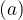$(a)$: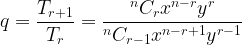$q = \dfrac{{{T_{r + 1}}}}{{{T_r}}} = \dfrac{{^n{C_r}{x^{n - r}}{y^r}}}{{^n{C_{r - 1}}{x^{n - r + 1}}{y^{r - 1}}}}$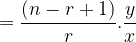$= \dfrac{{\left( {n - r + 1} \right)}}{r}.\dfrac{y}{x}$
Observe that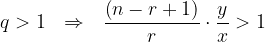$q > 1 \,\,\,\, \Rightarrow \,\,\,\, \dfrac{{\left( {n - r + 1} \right)}}{r} \cdot \dfrac{y}{x} > 1$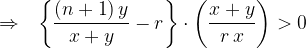$\Rightarrow \,\,\,\, \left\{ {\dfrac{{\left( {n + 1} \right)y}}{{x + y}} - r} \right\} \cdot \left( {\dfrac{{x + y}}{{r\,x}}} \right) > 0$
If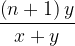$\dfrac{{\left( {n + 1} \right)y}}{{x + y}}$ is an integer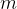$m$, which must lie in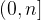$(0,n]$, we see that there are two greatest terms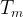${T_m}$ and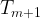${T_{m + 1}}$. (Why). Here’s the explanation:
We have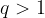$q > 1$ for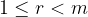$1 \le r < m$ and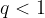$q < 1$ for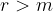$r > m$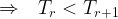$\Rightarrow \,\,\,\, {T_r} < {T_{r + 1}}$ for$1 \le r < m$ and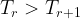${T_r} > {T_{r + 1}}$ for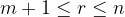$m + 1 \le r \le n$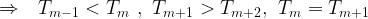$\Rightarrow \,\,\,\, {T_{m - 1}} < {T_m}\,\,,\,\,{T_{m + 1}} > {T_{m + 2}},\,\,{T_m} = {T_{m + 1}}$$\Rightarrow \,\,\,\, {T_m}\,\,{\rm{and}}\,\,{T_{m + 1}}$ are the two greatest terms
Now, if$\dfrac{{\left( {n + 1} \right)y}}{{x + y}}$ is a non-integer, assume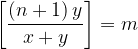$\left[ {\dfrac{{\left( {n + 1} \right)y}}{{x + y}}} \right] = m$.
We now have$q > 1$ for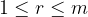$1 \le r \le m$ and$q < 1$ for$r > m$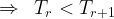$\Rightarrow \,\,\, {T_r} < {T_{r + 1}}$ for$1 \le r \le m$ and${T_r} > {T_{r + 1}}$ for$r > m$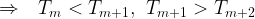$\Rightarrow \,\,\,\, {T_m} < {T_{m + 1}},\,\,{T_{m + 1}} > {T_{m + 2}}$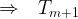$\Rightarrow \,\,\,\, {T_{m + 1}}$ is the greatest term